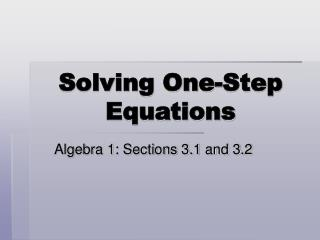DownloadDownload PresentationSolving One-Step Equations

# Solving One-Step Equations

Download Presentation## Solving One-Step Equations

- - - - - - - - - - - - - - - - - - - - - - - - - - - E N D - - - - - - - - - - - - - - - - - - - - - - - - - - -
##### Presentation Transcript

1. Solving One-Step Equations Algebra 1: Sections 3.1 and 3.2

2. Properties of Equality • The theory of equation solving is based on the Properties of Equality. • We start with something that is true, the equation. • By using the Properties of Equality, we simplify the equation into a simpler and simpler equation. • Eventually, we end up with an equation that looks like x = n, where n is the solution to the equation.

3. Properties of Equality • The Reflexive Property of Equality states that a number is equal to itself: a = a. • The Symmetric Property of Equality states that the order in which you write the equation does not matter:if a = b, then b = a. • The Transitive Property of Equality states that two things equal to the same thing are equal to each other:if a = b, and b = c, then a = c. • The Substitution Property of Equality states that two things equal to each other can be substituted into other expressions for each other:if a = b, then a may be substituted in for b in any expression where b appears.

4. Properties of Equality • The Addition Property of Equality states:if a = b, then a + c = b + c. • The Subtraction Property of Equality states:if a = b, then a – c = b – c. • The Multiplication Property of Equality states:if a = b, then ac = bc. • The Division Property of Equality states:if a = b, and c ≠ 0, then a ÷ c = b ÷ c.

5. Using the Properties of Equality to Solve Equations • Solve: x + 5 = -8 • Solution • So: x + 5 – 5 = -8 – 5 (Subt. Prop. Eq.) • And x + 5 – 5 = x + 0 (Inv. Prop. Add.) • And x + 0 = x (Id. Prop. Add.) • And -8 – 5 = -8 + (-5) = -13, (Addition) • So: x = -13 (Subs. Prop. Eq.)

6. Using the Properties of Equality to Solve Equations • Solve: x + 9 = 3 • Solution • So: x + 9 – 9 = 3 – 9 (Subt. Prop. Eq.) • And x + 9 – 9 = x + 0 (Inv. Prop. Add.) • And x + 0 = x (Id. Prop. Add.) • And 3 – 9 = 3 + (-9) = -6, (Addition) • So: x = -6 (Subs. Prop. Eq.)

7. Using the Properties of Equality to Solve Equations • Solve: x – 4 = -7 • Solution • So: x – 4 + 4 = -7 + 4 (Add. Prop. Eq.) • And x – 4 + 4 = x + 0 (Inv. Prop. Add.) • And x + 0 = x (Id. Prop. Add.) • And -7 + 4 = -3, (Addition) • So: x = -3 (Subs. Prop. Eq.)

8. Using the Properties of Equality to Solve Equations • Solve: x – 14 = -2 • Solution • So: x – 14 + 14 = -2 + 14 (Add. Prop. Eq.) • And x – 14 + 14 = x + 0 (Inv. Prop. Add.) • And x + 0 = x (Id. Prop. Add.) • And -2 + 14 = 12, (Addition) • So: x = 12 (Subs. Prop. Eq.)

9. Using the Properties of Equality to Solve Equations • Solve: • Solution • So: (Add. Prop. Eq.) • And (Inv. Prop. Add.) • And x + 0 = x (Id. Prop. Add.) • And (Addition) • So: (Subs. Prop. Eq.)

10. Using the Properties of Equality to Solve Equations • Solve: -3x = 12 • Solution • So: -3x ÷ (-3) = 12 ÷ (-3) (Div. Prop. Eq.) • And -3x ÷ (-3) = = 1x (Division) • And 1x = x (Id. Prop. Mult.) • And 12 ÷ (-3) = = -4, (Division) • So: x = -4 (Subs. Prop. Eq.)

11. Using the Properties of Equality to Solve Equations • Solve: • Solution • So: (Mult. Prop. Eq.) • And (Multiplication) • And 1x = x (Id. Prop. Mult.) • And (Multiplication) • So (Subs. Prop. Eq.)

12. Writing Linear Equations • Your bank account has \$764.77 in it. You withdraw just enough money to pay for a new pair of shoes. You then have \$688.24 left in the account. Write a linear equation to describe the situation. • Solution • We started with \$764.77 and then took some out and ended with \$688.24: • So the equation is 764.77 – x = 688.24.

13. Writing Linear Equations • A quadrilateral has sides of length 12, 16, 24 and x. The perimeter of the quadrilateral is 62. Write a linear equation that you could use to solve for x. • Solution • The lengths we know add up to 52. The total perimeter is 62. • The equation is 52 + x = 62.

14. Writing Linear Equations • A rectangle has sides of length 8 and x. The area of the quadrilateral is 56. Write a linear equation that you could use to solve for x. • Solution • The area of a rectangle is bh. • The equation is 8x = 56.